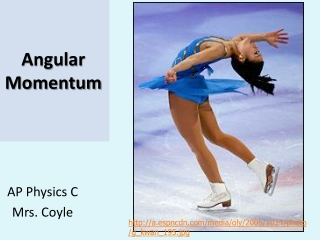DownloadDownload PresentationAngular Momentum

# Angular Momentum

Télécharger la présentation## Angular Momentum

- - - - - - - - - - - - - - - - - - - - - - - - - - - E N D - - - - - - - - - - - - - - - - - - - - - - - - - - -
##### Presentation Transcript

1. Angular Momentum AP Physics C Mrs. Coyle http://a.espncdn.com/media/oly/2005/1024/photo/g_kwan_195.jpg

2. Remember: Torque t=r┴ x F = rFsinf

3. Cross Product • The cross product of two vectors AxB is a third vector that is perpendicular to the plane of A and B (according to the right hand rule).

4. Properties of the Cross Product 1) AxB=-BxA (not commutative) 2) AxA=0 (or if A is // B, AxB=0) 3)AxB=AB, when A _|_ B

5. Properties of the Cross Product, Cont’d 4) Ax(B+C)=AxB +AxC (distributive) 5)

6. Properties of Cross Products of Unit Vectors ixi=0 jxj=0 kxk=0 ixj=k jxk=I kxi=j

7. Example #3 Two vectors are given by A=-3i+4j and B=2i+3j. Find: a)AxB b) the angle between A and B Ans: a)-17.0k, b)70.6o

8. Angular Momentum, L For a particle of mass m at position r and linear momentum p.

9. Angular Momentum, L= r x p The instantaneous angular momentum of a particle relative to the origin is defined as the cross product of the particle’s instantaneous position vector r and its instantaneous linear momentum p L = r x p

10. Angular Momentum L = r mv sin f • is the angle between r and p . Remember: p=mv

11. Rotational Analog of Newton’s Second Law • St and L must be measured about the same origin. • For origin fixed in an inertial frame.

12. Units of Angular Momentum SI units: (kg.m2)/ s

13. Direction of Angular Momentum • The magnitude and direction of L depend on the choice of origin • The direction of L is perpendicular to the plane formed by r and p.

14. Example: Uniform Circular Motion A particle in uniform circular motion has a constant angular momentum about an axis through the center of its path.

15. Angular Momentum of a System of Particles: • The vector sum of the angular momenta of the individual particles Ltot = L1 + L2 + …+ Ln = SLi

16. Note: • Any torques associated with the internal forces acting in a system of particles are zero. • So:

17. Resultant Torque about the CM • The resultant torque acting on a system about an axis through the center of mass equals the time rate of change of angular momentum of the system regardless of the motion of the center of mass (even if the center of mass is accelerating).

18. Ex #11 A light rod 1.00m in length joins two particles, with masses 4kg and 3kg at its ends. The combination moves in the xy plane about a pivot the center of the rod, counterclockwise. Determine the angular momentum of the system about the origin when the speed of each particle is 5m/s. Ans: 17.5k kg m2 /s